# Introduction to Linear Inequalities

Go back to  'Linear-Equations'

When one expression is given to be greater than or less than another expression, we have an inequation.For example, consider:

$2x + 3 > 7$

This is an example of an inequation in one variable. The solution to this inequation will be the set of all values of x for which this inequation is satisfied, that is, the left side is greater than the right side. The solution in this case is simple to evaluate:

$2x > 7 - 3\,\,\,\Rightarrow \;\;2x > 4\,\,\, \Rightarrow \;\;x > 2$

Thus, all values of greater than 2 will satisfy this inequality, because for all values of greater than 2, the term $$2x + 3$$ will be greater than 7.

Now, consider the inequation

$x + 2y > 3$

This is an example of an inequation in two variables. The solution to this inequation will be the set of all pairs of values for x and y such that the expression $$x + 2y$$ is greater than 3.For example, one possible solution is $$x =3,\;y = 1,$$ because

$\left( 3 \right) +2\left( 1 \right) = 5 > 3$

Note that this is just one possible solution. The solution to the inequation as a whole will be all pairs of values which satisfy the inequation.

The two examples considered above have strict inequalities: this means that the two sides can never be equal. However, we can also have inequations which don’t have strict inequalities. For example,

$\begin{array}{l} -3x + 2 \ge 5\\x - 4y \le \sqrt 2 \end{array}$

are linear inequations which are not strict, because the two sides in each inequation can also be equal.

As we have seen, a linear inequation in one variable involves an inequality between two linear expressions, or between a linear expression and a constant, where there is only one variable involved. Some examples:

$\begin{array}{l}x +7 < \sqrt 2 \\ - 3 - \sqrt 3 x \ge 10\\3x + 1 \le \pi x + 2\\1 - 10x > 2+ 11x\end{array}$

Every linear inequation in one variable can be simplified to the following general forms:

$\begin{array}{l}ax +b > 0 & {\rm{or}} & ax + b \ge 0\\ax + b < 0 & {\rm{or}}& ax + b \le 0\end{array}$

a and b are arbitrary real constants, and a is not equal to 0.

How do we solve a linear inequation, that is, how do we find the solution to a linear inequation? Consider the following inequation:

$3x + 1 \le 7$

We proceed as follows:

$3x \le 7 - 1\,\,\,\Rightarrow \;\;\;3x \le 6\,\,\, \Rightarrow \;\;\;x \le 2$

Now, we want to represent this solution set on a number line. Thus, we simply highlight that part of the number line lying to the left of 2: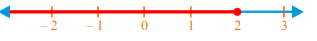We see that any number lying on the red part of the number line will satisfy this inequation, and so it is a part of the solution set for this inequation. Note that we have drawn a solid dot exactly at the point 2. This is to indicate that 2 is also a part of the solution set.

Now, consider the following inequation:$4x > - 3x +21$

We first solve this inequation algebraically:

$4x + 3x >21\,\,\, \Rightarrow \;\;\;7x > 21\,\,\, \Rightarrow \;\;\;x > 3$

Now, we plot the solution set on a number line: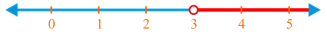Any point lying on the red part of the number line will satisfy this inequation. Note that in this case, we have drawn a hollow dot at the point 3. This is to indicate that 3 is not a part of the solution set (this is because the given inequation had a strict inequality).

Consider the following inequation:

$- 2x + 3 > 5$

Suppose that we solve this inequation as follows:

$- 2x > 5 -3\,\,\, \Rightarrow \;\;\; - 2x > 2\,\,\, \Rightarrow \;\;\;x > - 1$

Is this solution correct? No, it is not! Let us try to understand why. Let us pick an arbitrary number in this solution set, say $$x = 2,$$ and see whether it satisfies the original inequation. We have

$- 2\left( 2 \right)+ 3 = - 4 + 3 = - 1$

This is not greater than 5, and thus x equal to 2 does not satisfy the original inequation, which means that the solution set $$x > - 1$$ is incorrect. Where did we go wrong?

To understand that, consider the following  inequation:

$- x > 1$

Can we multiply –1 on both sides and say the following?

$\begin{array}{l}\left({ - 1} \right) \times \left( { - x} \right) > \left( { - 1} \right) \times1\\ \Rightarrow \;\;\;x > - 1\end{array}$

No! This is incorrect. If –xis greater than 1, this cannot mean that x is greater than –1. In fact,what this will actually mean is that x is less than –1. Only then can the negative of x be greater than 1. Take your time and reflect on this argument in detail.

Thus, when we multiplied the inequation by –1 on both sides, we should actually have reversed the direction of the inequality:

$\begin{array}{l} - x> 1\\ \Rightarrow \;\;\;\left( { - 1} \right) \times \left( { - x} \right)< \left( { - 1} \right) \times 1\\ \Rightarrow \;\;\;x < -1\end{array}$

In fact, extending this line of reasoning, whenever you multiply two sides of an inequation by a negative number, the direction of the inequality will get reversed.

Let us summarize our discussion into the following rules:

(1) You can add or subtract any real number on both sides of an inequation:

$\begin{array}{l}x> a\\ \Rightarrow \;\;\;\left\{ \begin{array}{l}x + k > a + k\\x - k >a - k\end{array} \right.\end{array}$

(2) You can multiply any positive real number on both sides of an inequation:

$\begin{array}{l}x> a,\,\,\,k > 0\\ \Rightarrow \;\;kx > ka\end{array}$

(3) If you multiply a negative real number on both sides of an inequation, the direction of the inequality  will get reversed:

$\begin{array}{l}x> a,\,\,\,k < 0\\ \Rightarrow \;\;\;kx < ka\end{array}$

Example 1: Solve the following inequation, and plot the solution set on a number line:

$2x - 5 > 3 - 7x$

Solution: We have:

$2x - 5 > 3 -7x\,\,\, \Rightarrow \;\;9x > 8\,\,\, \Rightarrow \;\;x > \frac{8}{9}$

The solution set is plotted below. Note the hollow dot carefully: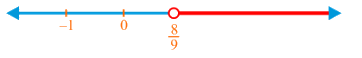Example 2: Solve the following inequation, and plot the solution set on a number line:

$- 2x - 39 \ge -15$

Solution: We have:

$\begin{array}{l} -2x - 39 \ge - 15\,\,\, \Rightarrow \;\; - 2x \ge 24\\ \Rightarrow \;\;2x \le - 24\,\,\, \Rightarrow \;\;x \le - 12\end{array}$

The solution set is plotted below. Note the solid dot at x equal to –12.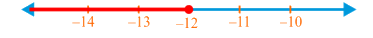Example 3: A number satisfies both the following linear inequations simultaneously:

$\begin{array}{l}2x +1 > 7\\ - 3x > - 18\end{array}$

How many integer values can take?

Solution: We will find the solution sets for the two inequations separately, and then find those values of which are common to both the solution sets.

### Inequality - 1

We have:

$2x + 1 > 7\,\,\,\Rightarrow \;\;\;2x > 6\,\,\, \Rightarrow \;\;\;x > 3$

### Inequality - 2

We have

$- 3x > -18\,\,\, \Rightarrow \;\;\;3x < 18\,\,\, \Rightarrow \;\;\;x < 6$

Now, for x to simultaneously satisfy both inequations, it must be greater than 3 as well as less than 6. Thus, the solution set for the two inequations taken together will be as follows: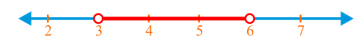We see that x can take two integer values: 4 and 5. Note that the solution set itself is infinite. can take any value between 3 and 6. However, there are only two integer values between 3 and 6.

Learn math from the experts and clarify doubts instantly

• Instant doubt clearing (live one on one)
• Learn from India’s best math teachers
• Completely personalized curriculum